# Frank Solutions for Class 9 Maths Chapter 4 Expansions

Frank Solutions for Class 9 Maths Chapter 4 Expansions has accurate answers designed by experienced teachers, which helps students in boosting exam preparation. The solutions, in a step by step manner, enable them to grasp the concepts easily. From the exam perspective, solving these problems on a daily basis, improves their conceptual knowledge. To know more about the concepts, students can access Frank Solutions for Class 9 Maths Chapter 4 Expansions PDF, from the links which are provided below.

Chapter 4 mainly deals with the problems based on Expansions. Students can analyse their mistakes by cross checking their answers, referring to these solutions given in PDF format. Revising the problems with the help of these solutions, students understand the various methods to solve the complex problems effortlessly.

## Frank Solutions for Class 9 Maths Chapter 4 Expansions Download PDF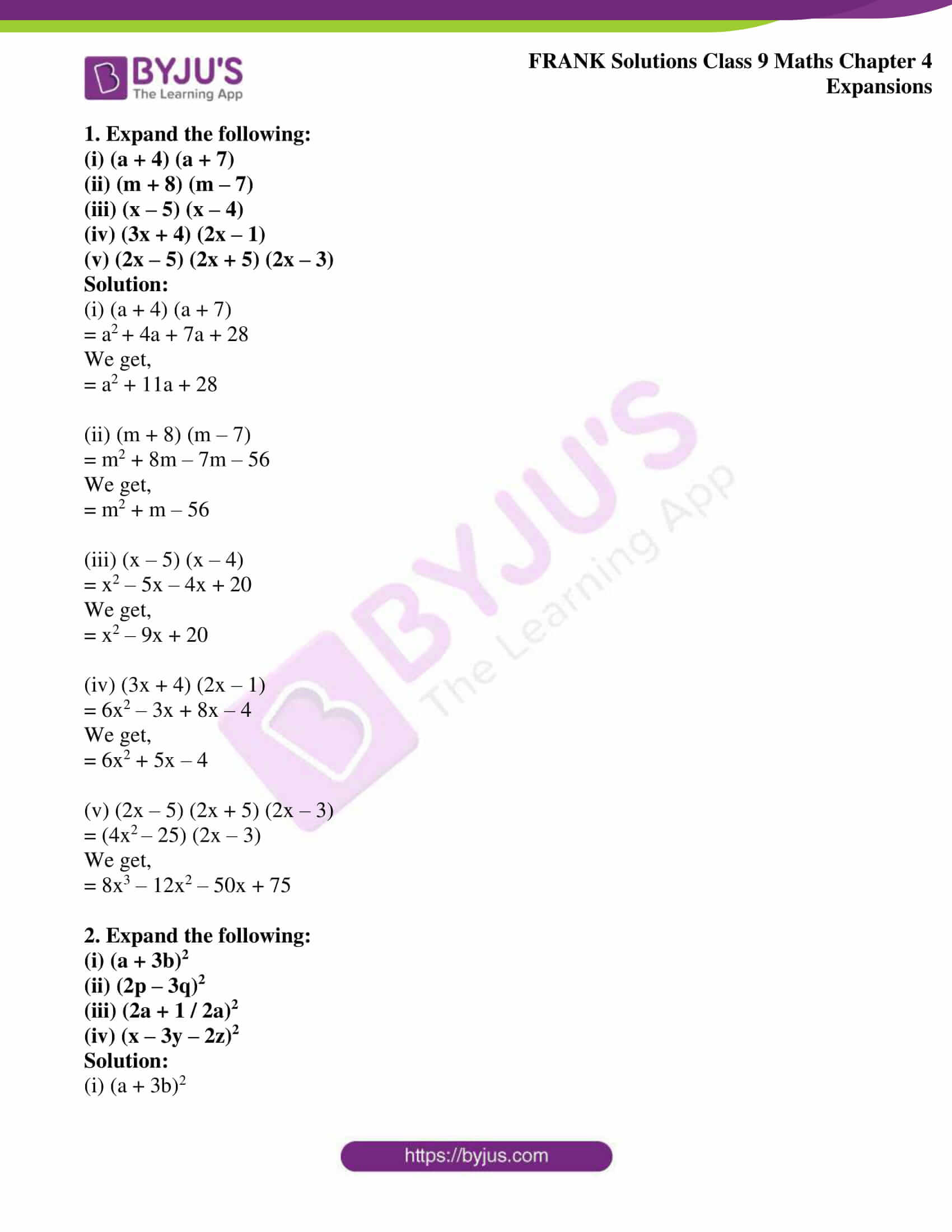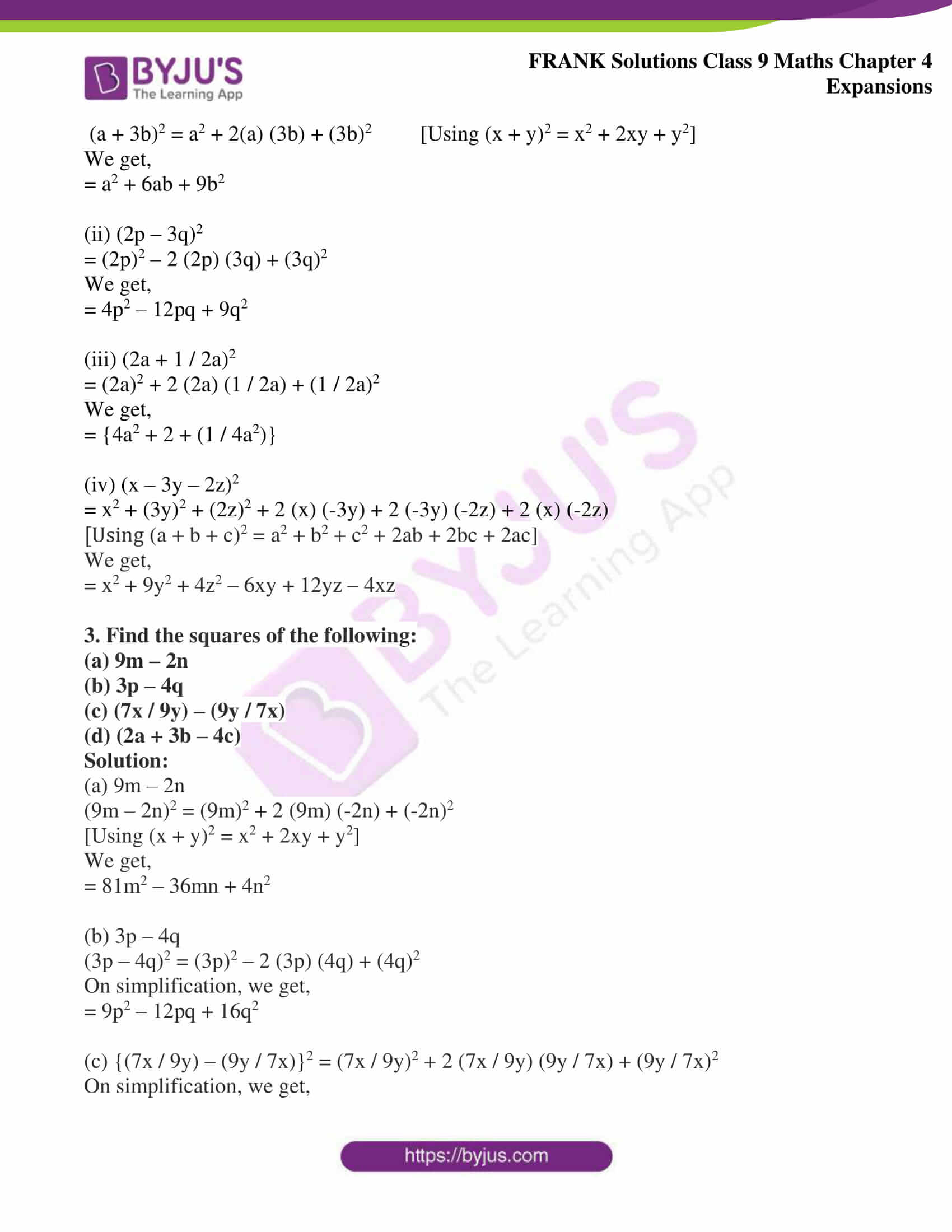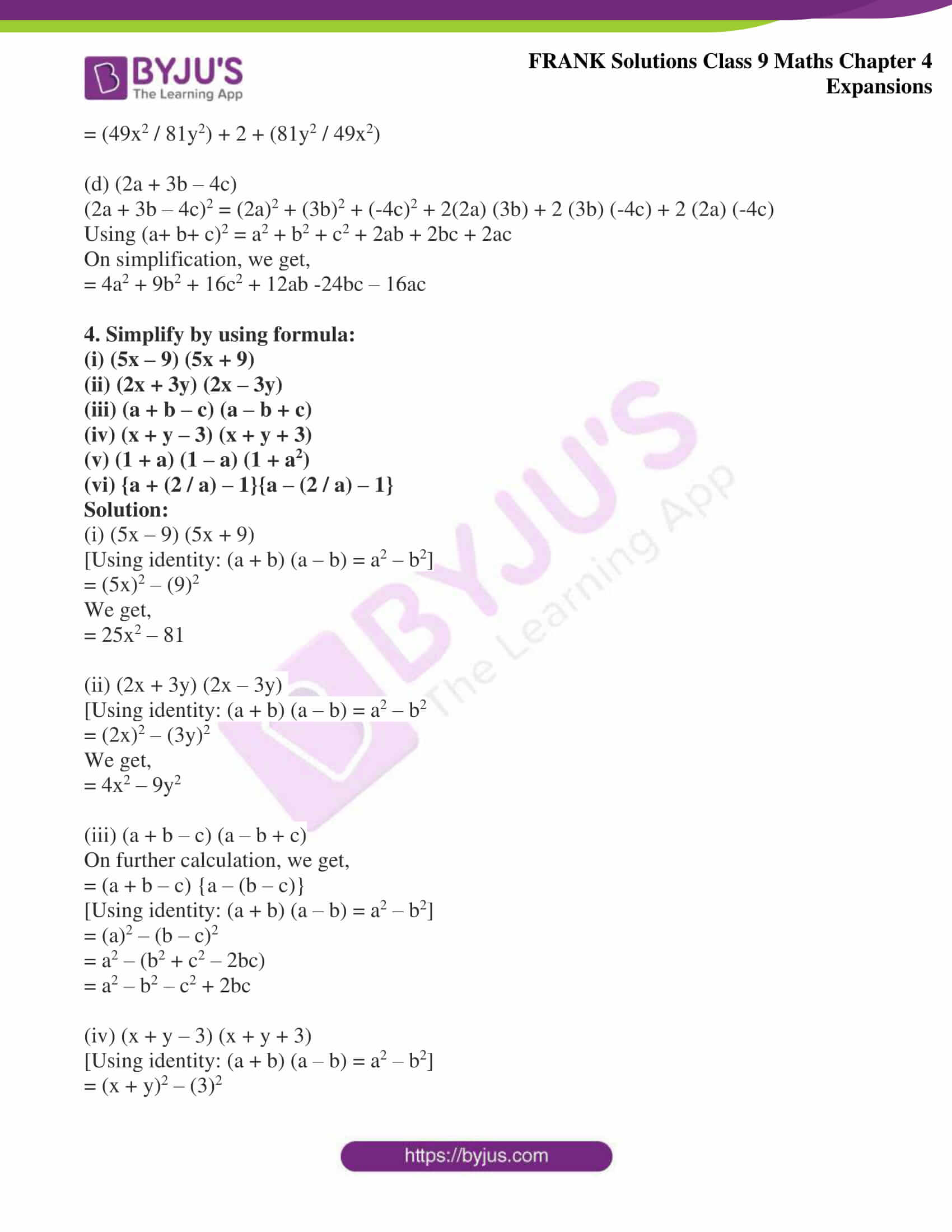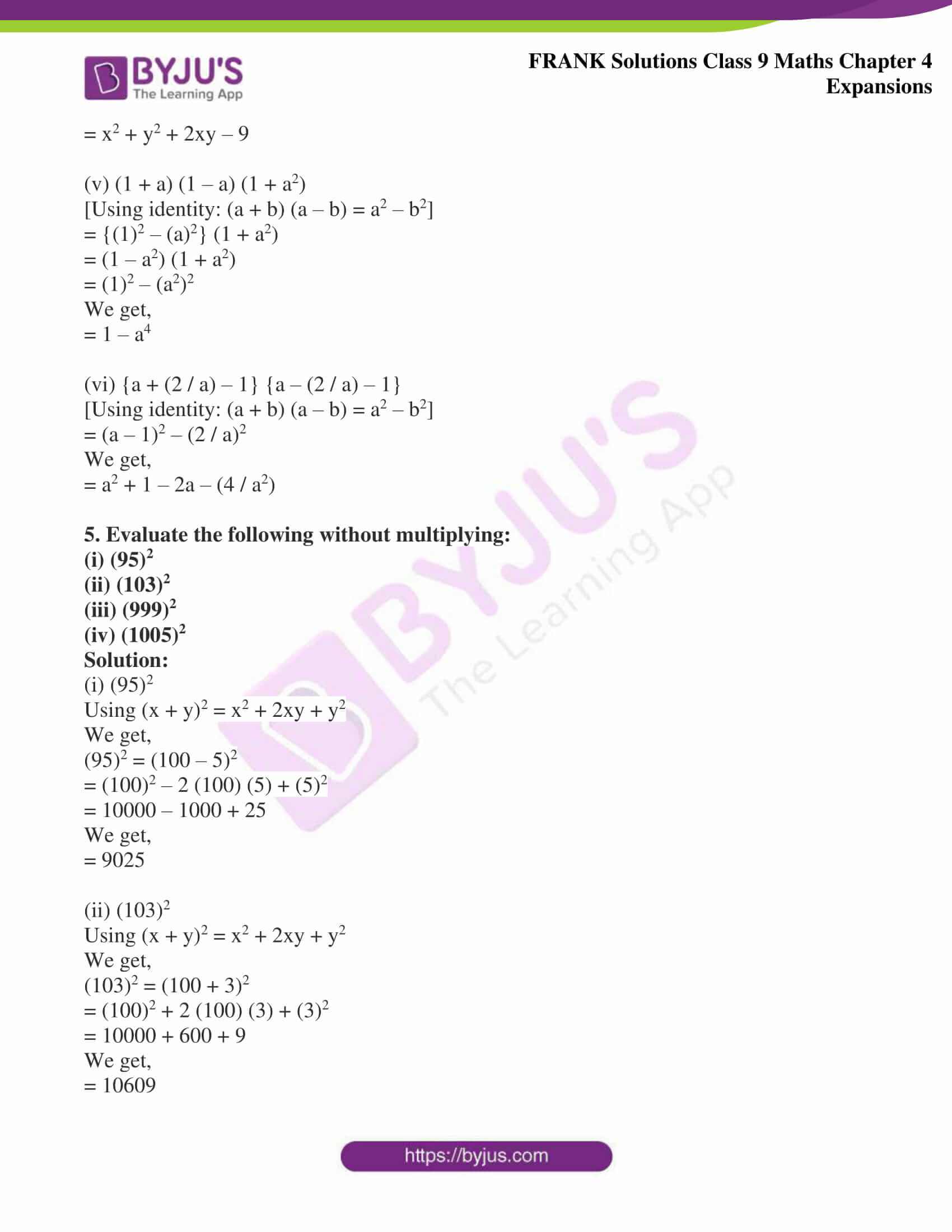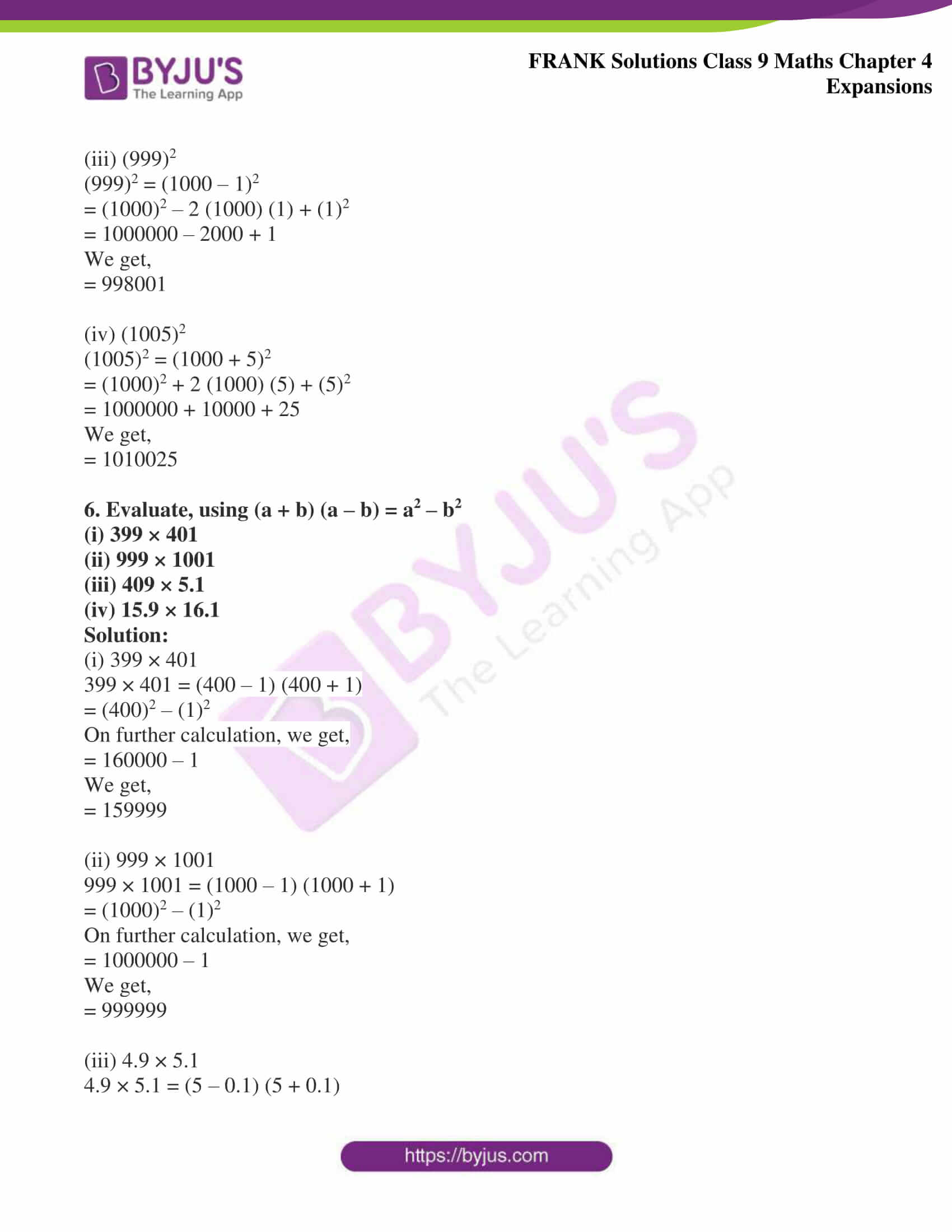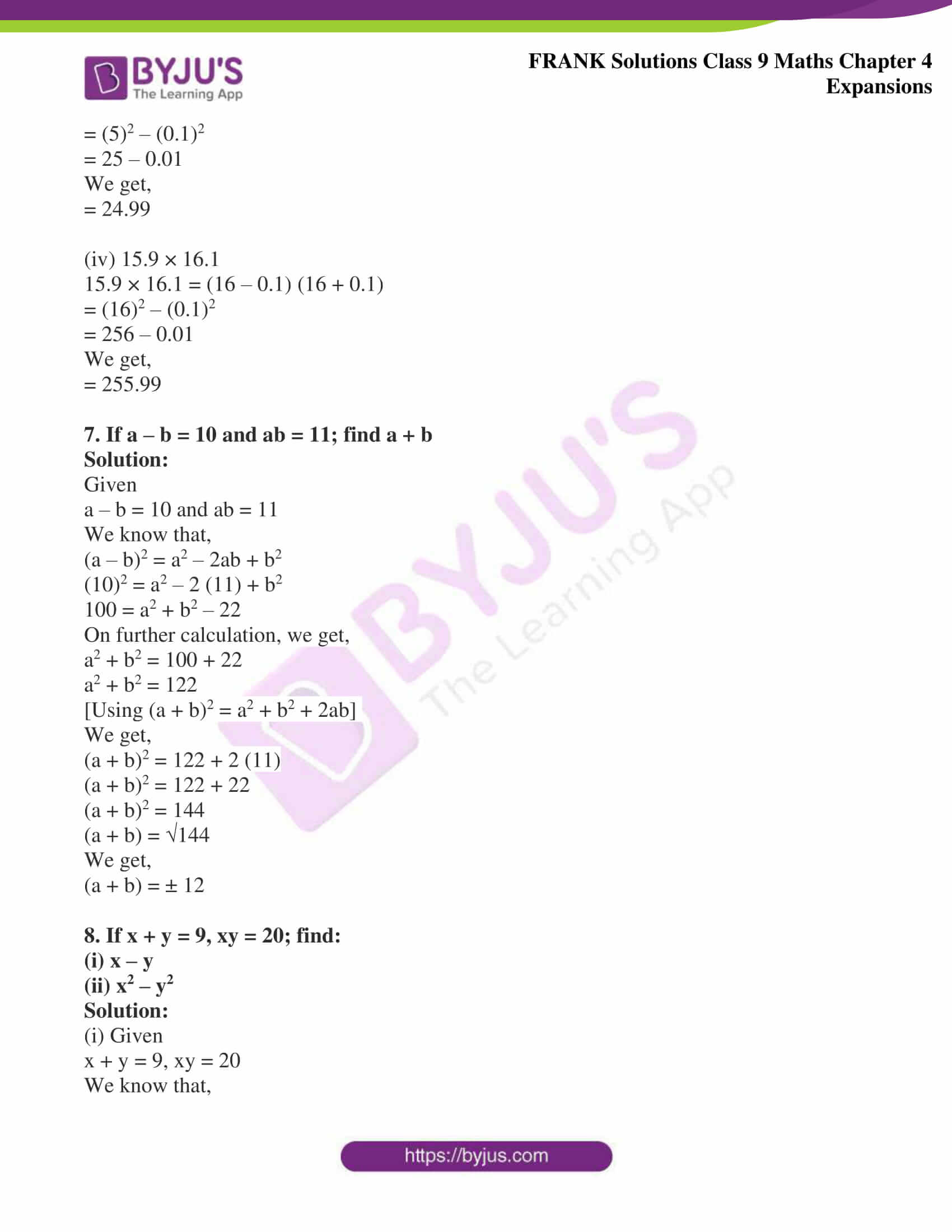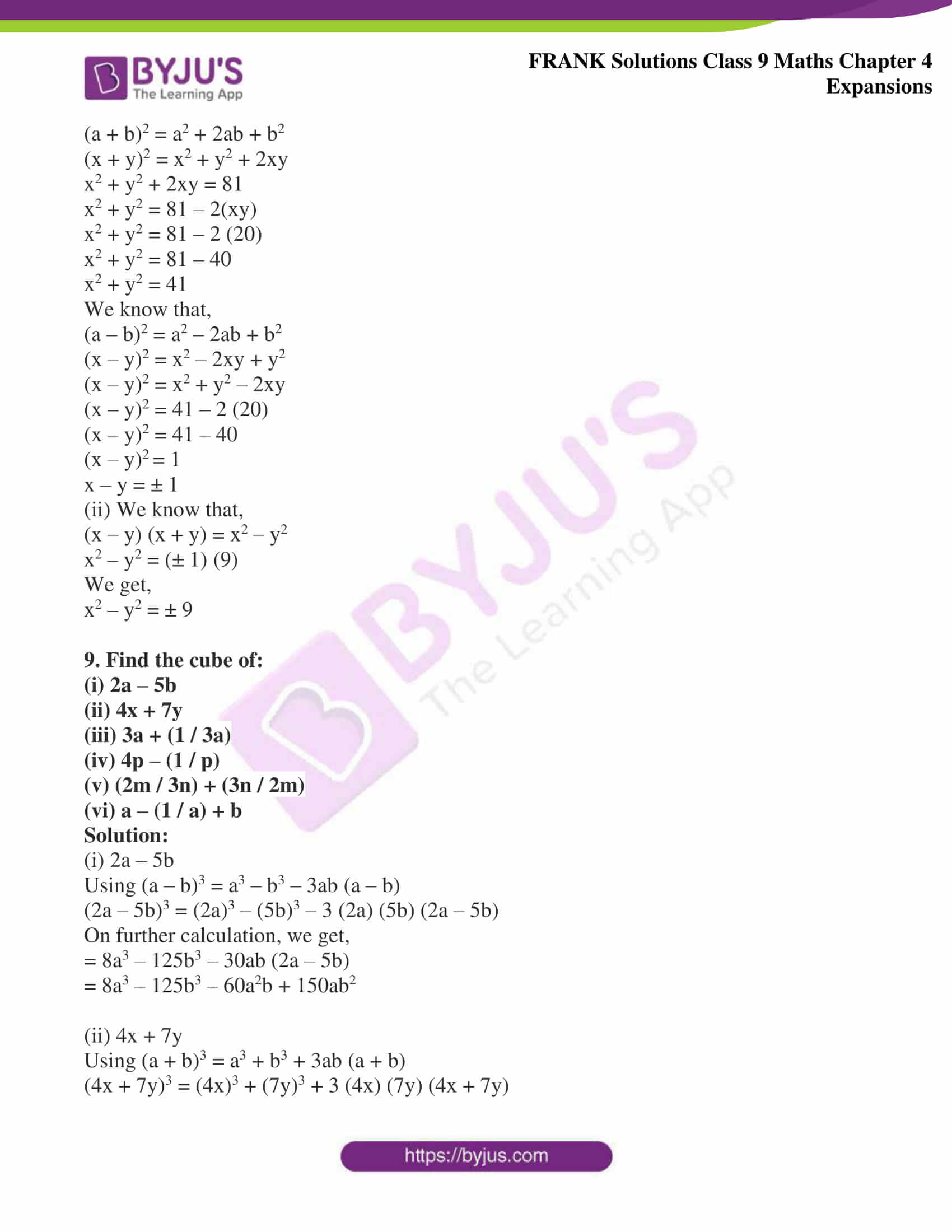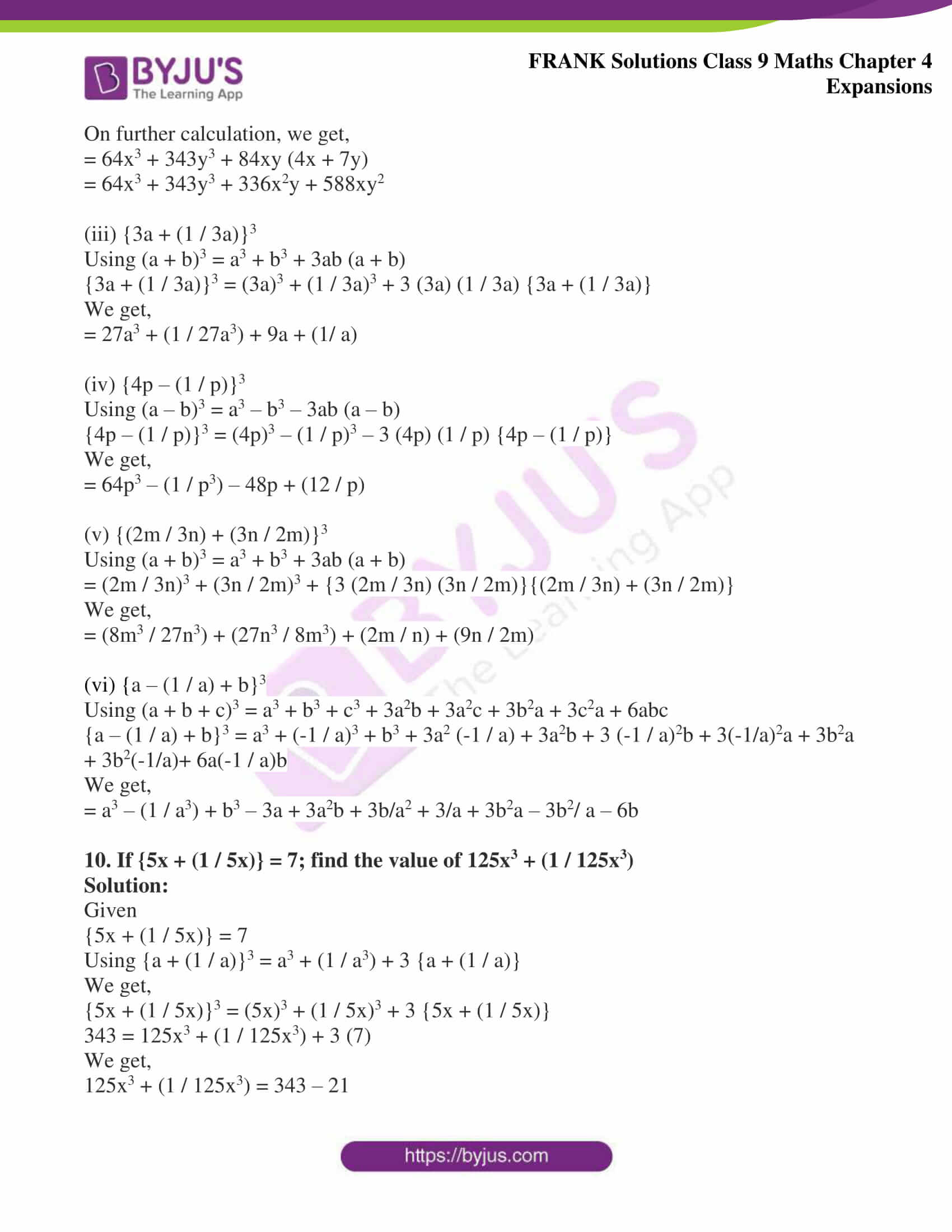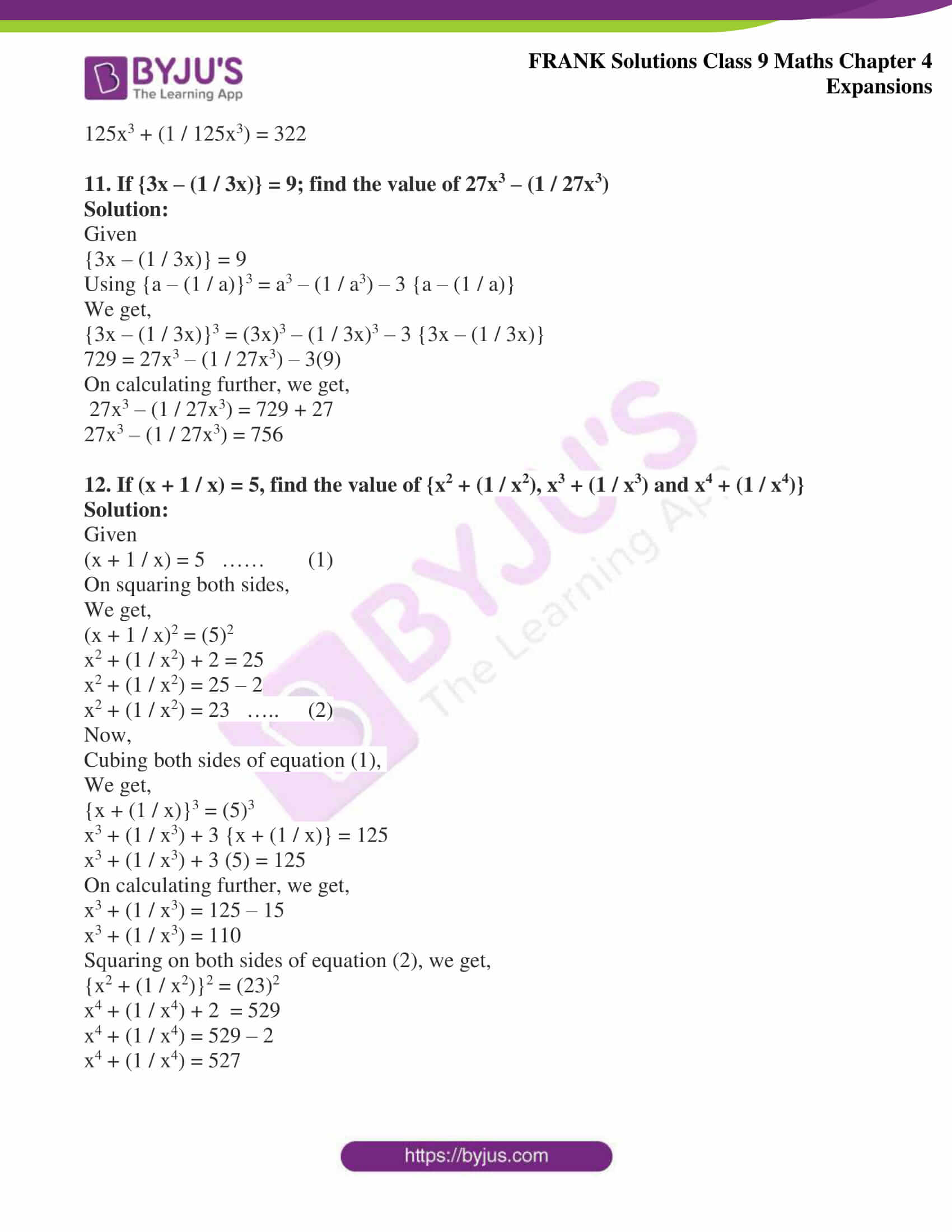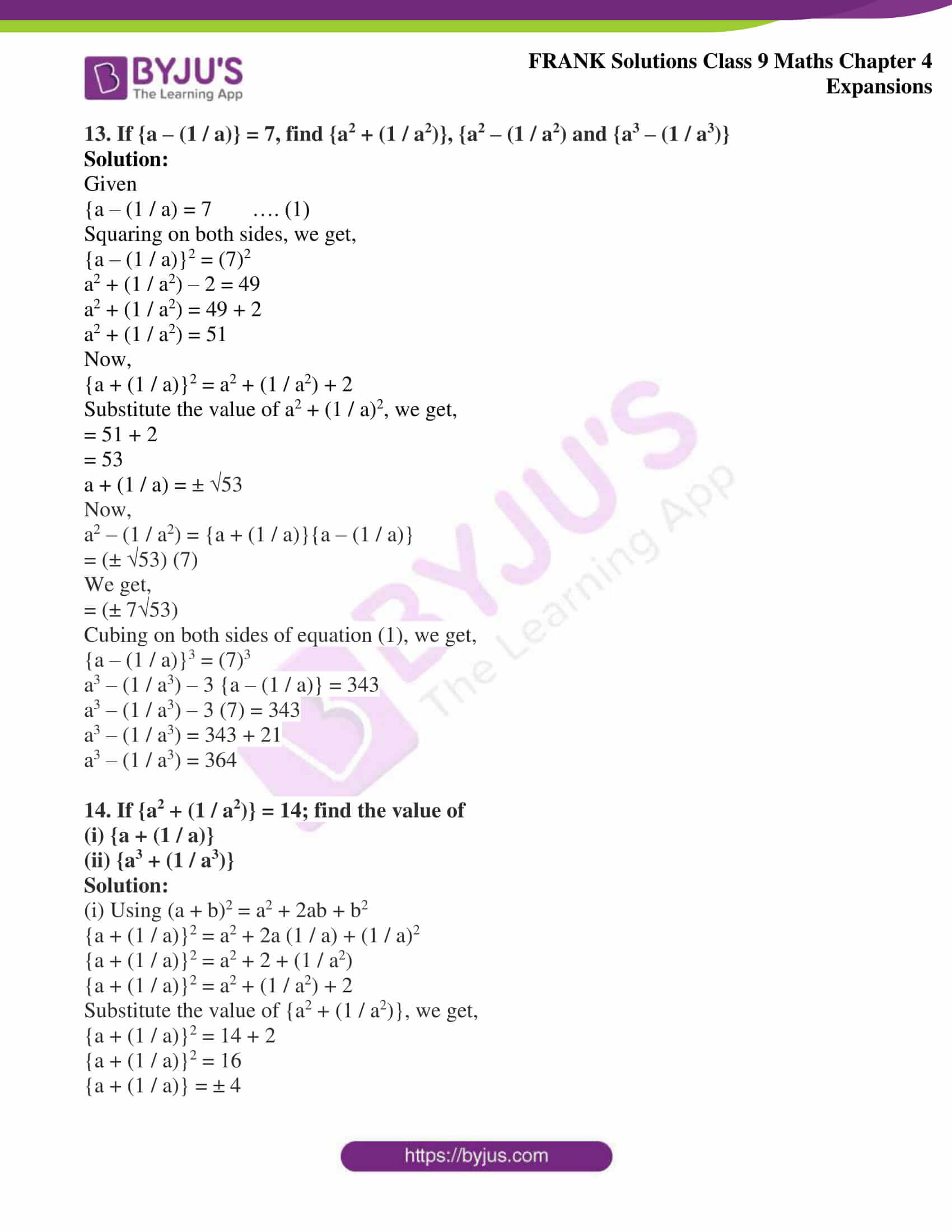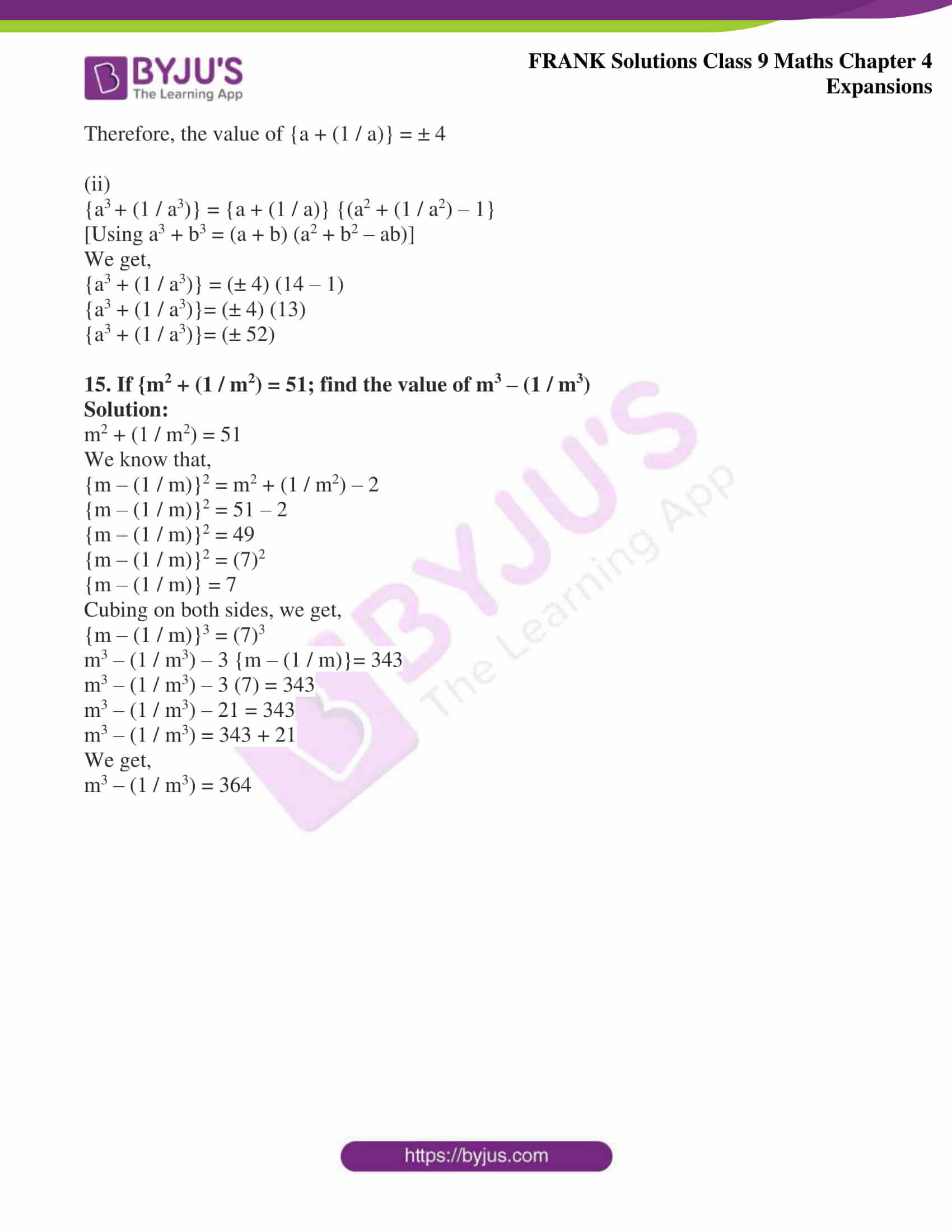## Access Frank Solutions for Class 9 Maths Chapter 4 Expansions

1. Expand the following:

(i) (a + 4) (a + 7)

(ii) (m + 8) (m – 7)

(iii) (x – 5) (x – 4)

(iv) (3x + 4) (2x – 1)

(v) (2x – 5) (2x + 5) (2x – 3)

Solution:

(i) (a + 4) (a + 7)

= a2 + 4a + 7a + 28

We get,

= a2 + 11a + 28

(ii) (m + 8) (m – 7)

= m2 + 8m – 7m – 56

We get,

= m2 + m – 56

(iii) (x – 5) (x – 4)

= x2 – 5x – 4x + 20

We get,

= x2 – 9x + 20

(iv) (3x + 4) (2x – 1)

= 6x2 – 3x + 8x – 4

We get,

= 6x2 + 5x – 4

(v) (2x – 5) (2x + 5) (2x – 3)

= (4x2 – 25) (2x – 3)

We get,

= 8x3 – 12x2 – 50x + 75

2. Expand the following:

(i) (a + 3b)2

(ii) (2p – 3q)2

(iii) (2a + 1 / 2a)2

(iv) (x – 3y – 2z)2

Solution:

(i) (a + 3b)2

(a + 3b)2 = a2 + 2(a) (3b) + (3b)2 [Using (x + y)2 = x2 + 2xy + y2]

We get,

= a2 + 6ab + 9b2

(ii) (2p – 3q)2

= (2p)2 – 2 (2p) (3q) + (3q)2

We get,

= 4p2 – 12pq + 9q2

(iii) (2a + 1 / 2a)2

= (2a)2 + 2 (2a) (1 / 2a) + (1 / 2a)2

We get,

= {4a2 + 2 + (1 / 4a2)}

(iv) (x – 3y – 2z)2

= x2 + (3y)2 + (2z)2 + 2 (x) (-3y) + 2 (-3y) (-2z) + 2 (x) (-2z)

[Using (a + b + c)2 = a2 + b2 + c2 + 2ab + 2bc + 2ac]

We get,

= x2 + 9y2 + 4z2 – 6xy + 12yz – 4xz

3. Find the squares of the following:

(a) 9m – 2n

(b) 3p – 4q

(c) (7x / 9y) – (9y / 7x)

(d) (2a + 3b – 4c)

Solution:

(a) 9m – 2n

(9m – 2n)2 = (9m)2 + 2 (9m) (-2n) + (-2n)2

[Using (x + y)2 = x2 + 2xy + y2]

We get,

= 81m2 – 36mn + 4n2

(b) 3p – 4q

(3p – 4q)2 = (3p)2 – 2 (3p) (4q) + (4q)2

On simplification, we get,

= 9p2 – 12pq + 16q2

(c) {(7x / 9y) – (9y / 7x)}2 = (7x / 9y)2 + 2 (7x / 9y) (9y / 7x) + (9y / 7x)2

On simplification, we get,

= (49x2 / 81y2) + 2 + (81y2 / 49x2)

(d) (2a + 3b – 4c)

(2a + 3b – 4c)2 = (2a)2 + (3b)2 + (-4c)2 + 2(2a) (3b) + 2 (3b) (-4c) + 2 (2a) (-4c)

Using (a+ b+ c)2 = a2 + b2 + c2 + 2ab + 2bc + 2ac

On simplification, we get,

= 4a2 + 9b2 + 16c2 + 12ab -24bc – 16ac

4. Simplify by using formula:

(i) (5x – 9) (5x + 9)

(ii) (2x + 3y) (2x – 3y)

(iii) (a + b – c) (a – b + c)

(iv) (x + y – 3) (x + y + 3)

(v) (1 + a) (1 – a) (1 + a2)

(vi) {a + (2 / a) – 1}{a – (2 / a) – 1}

Solution:

(i) (5x – 9) (5x + 9)

[Using identity: (a + b) (a – b) = a2 – b2]

= (5x)2 – (9)2

We get,

= 25x2 – 81

(ii) (2x + 3y) (2x – 3y)

[Using identity: (a + b) (a – b) = a2 – b2

= (2x)2 – (3y)2

We get,

= 4x2 – 9y2

(iii) (a + b – c) (a – b + c)

On further calculation, we get,

= (a + b – c) {a – (b – c)}

[Using identity: (a + b) (a – b) = a2 – b2]

= (a)2 – (b – c)2

= a2 – (b2 + c2 – 2bc)

= a2 – b2 – c2 + 2bc

(iv) (x + y – 3) (x + y + 3)

[Using identity: (a + b) (a – b) = a2 – b2]

= (x + y)2 – (3)2

= x2 + y2 + 2xy – 9

(v) (1 + a) (1 – a) (1 + a2)

[Using identity: (a + b) (a – b) = a2 – b2]

= {(1)2 – (a)2} (1 + a2)

= (1 – a2) (1 + a2)

= (1)2 – (a2)2

We get,

= 1 – a4

(vi) {a + (2 / a) – 1} {a – (2 / a) – 1}

[Using identity: (a + b) (a – b) = a2 – b2]

= (a – 1)2 – (2 / a)2

We get,

= a2 + 1 – 2a – (4 / a2)

5. Evaluate the following without multiplying:

(i) (95)2

(ii) (103)2

(iii) (999)2

(iv) (1005)2

Solution:

(i) (95)2

Using (x + y)2 = x2 + 2xy + y2

We get,

(95)2 = (100 – 5)2

= (100)2 – 2 (100) (5) + (5)2

= 10000 – 1000 + 25

We get,

= 9025

(ii) (103)2

Using (x + y)2 = x2 + 2xy + y2

We get,

(103)2 = (100 + 3)2

= (100)2 + 2 (100) (3) + (3)2

= 10000 + 600 + 9

We get,

= 10609

(iii) (999)2

(999)2 = (1000 – 1)2

= (1000)2 – 2 (1000) (1) + (1)2

= 1000000 – 2000 + 1

We get,

= 998001

(iv) (1005)2

(1005)2 = (1000 + 5)2

= (1000)2 + 2 (1000) (5) + (5)2

= 1000000 + 10000 + 25

We get,

= 1010025

6. Evaluate, using (a + b) (a – b) = a2 – b2

(i) 399 × 401

(ii) 999 × 1001

(iii) 409 × 5.1

(iv) 15.9 × 16.1

Solution:

(i) 399 × 401

399 × 401 = (400 – 1) (400 + 1)

= (400)2 – (1)2

On further calculation, we get,

= 160000 – 1

We get,

= 159999

(ii) 999 × 1001

999 × 1001 = (1000 – 1) (1000 + 1)

= (1000)2 – (1)2

On further calculation, we get,

= 1000000 – 1

We get,

= 999999

(iii) 4.9 × 5.1

4.9 × 5.1 = (5 – 0.1) (5 + 0.1)

= (5)2 – (0.1)2

= 25 – 0.01

We get,

= 24.99

(iv) 15.9 × 16.1

15.9 × 16.1 = (16 – 0.1) (16 + 0.1)

= (16)2 – (0.1)2

= 256 – 0.01

We get,

= 255.99

7. If a – b = 10 and ab = 11; find a + b

Solution:

Given

a – b = 10 and ab = 11

We know that,

(a – b)2 = a2 – 2ab + b2

(10)2 = a2 – 2 (11) + b2

100 = a2 + b2 – 22

On further calculation, we get,

a2 + b2 = 100 + 22

a2 + b2 = 122

[Using (a + b)2 = a2 + b2 + 2ab]

We get,

(a + b)2 = 122 + 2 (11)

(a + b)2 = 122 + 22

(a + b)2 = 144

(a + b) = √144

We get,

(a + b) = ± 12

8. If x + y = 9, xy = 20; find:

(i) x – y

(ii) x2 – y2

Solution:

(i) Given

x + y = 9, xy = 20

We know that,

(a + b)2 = a2 + 2ab + b2

(x + y)2 = x2 + y2 + 2xy

x2 + y2 + 2xy = 81

x2 + y2 = 81 – 2(xy)

x2 + y2 = 81 – 2 (20)

x2 + y2 = 81 – 40

x2 + y2 = 41

We know that,

(a – b)2 = a2 – 2ab + b2

(x – y)2 = x2 – 2xy + y2

(x – y)2 = x2 + y2 – 2xy

(x – y)2 = 41 – 2 (20)

(x – y)2 = 41 – 40

(x – y)2 = 1

x – y = ± 1

(ii) We know that,

(x – y) (x + y) = x2 – y2

x2 – y2 = (± 1) (9)

We get,

x2 – y2 = ± 9

9. Find the cube of:

(i) 2a – 5b

(ii) 4x + 7y

(iii) 3a + (1 / 3a)

(iv) 4p – (1 / p)

(v) (2m / 3n) + (3n / 2m)

(vi) a – (1 / a) + b

Solution:

(i) 2a – 5b

Using (a – b)3 = a3 – b3 – 3ab (a – b)

(2a – 5b)3 = (2a)3 – (5b)3 – 3 (2a) (5b) (2a – 5b)

On further calculation, we get,

= 8a3 – 125b3 – 30ab (2a – 5b)

= 8a3 – 125b3 – 60a2b + 150ab2

(ii) 4x + 7y

Using (a + b)3 = a3 + b3 + 3ab (a + b)

(4x + 7y)3 = (4x)3 + (7y)3 + 3 (4x) (7y) (4x + 7y)

On further calculation, we get,

= 64x3 + 343y3 + 84xy (4x + 7y)

= 64x3 + 343y3 + 336x2y + 588xy2

(iii) {3a + (1 / 3a)}3

Using (a + b)3 = a3 + b3 + 3ab (a + b)

{3a + (1 / 3a)}3 = (3a)3 + (1 / 3a)3 + 3 (3a) (1 / 3a) {3a + (1 / 3a)}

We get,

= 27a3 + (1 / 27a3) + 9a + (1/ a)

(iv) {4p – (1 / p)}3

Using (a – b)3 = a3 – b3 – 3ab (a – b)

{4p – (1 / p)}3 = (4p)3 – (1 / p)3 – 3 (4p) (1 / p) {4p – (1 / p)}

We get,

= 64p3 – (1 / p3) – 48p + (12 / p)

(v) {(2m / 3n) + (3n / 2m)}3

Using (a + b)3 = a3 + b3 + 3ab (a + b)

= (2m / 3n)3 + (3n / 2m)3 + {3 (2m / 3n) (3n / 2m)}{(2m / 3n) + (3n / 2m)}

We get,

= (8m3 / 27n3) + (27n3 / 8m3) + (2m / n) + (9n / 2m)

(vi) {a – (1 / a) + b}3

Using (a + b + c)3 = a3 + b3 + c3 + 3a2b + 3a2c + 3b2a + 3c2a + 6abc

{a – (1 / a) + b}3 = a3 + (-1 / a)3 + b3 + 3a2 (-1 / a) + 3a2b + 3 (-1 / a)2b + 3(-1/a)2a + 3b2a + 3b2(-1/a)+ 6a(-1 / a)b

We get,

= a3 – (1 / a3) + b3 – 3a + 3a2b + 3b/a2 + 3/a + 3b2a – 3b2/ a – 6b

10. If {5x + (1 / 5x)} = 7; find the value of 125x3 + (1 / 125x3)

Solution:

Given

{5x + (1 / 5x)} = 7

Using {a + (1 / a)}3 = a3 + (1 / a3) + 3 {a + (1 / a)}

We get,

{5x + (1 / 5x)}3 = (5x)3 + (1 / 5x)3 + 3 {5x + (1 / 5x)}

343 = 125x3 + (1 / 125x3) + 3 (7)

We get,

125x3 + (1 / 125x3) = 343 – 21

125x3 + (1 / 125x3) = 322

11. If {3x – (1 / 3x)} = 9; find the value of 27x3 – (1 / 27x3)

Solution:

Given

{3x – (1 / 3x)} = 9

Using {a – (1 / a)}3 = a3 – (1 / a3) – 3 {a – (1 / a)}

We get,

{3x – (1 / 3x)}3 = (3x)3 – (1 / 3x)3 – 3 {3x – (1 / 3x)}

729 = 27x3 – (1 / 27x3) – 3(9)

On calculating further, we get,

27x3 – (1 / 27x3) = 729 + 27

27x3 – (1 / 27x3) = 756

12. If (x + 1 / x) = 5, find the value of {x2 + (1 / x2), x3 + (1 / x3) and x4 + (1 / x4)}

Solution:

Given

(x + 1 / x) = 5 …… (1)

On squaring both sides,

We get,

(x + 1 / x)2 = (5)2

x2 + (1 / x2) + 2 = 25

x2 + (1 / x2) = 25 – 2

x2 + (1 / x2) = 23 ….. (2)

Now,

Cubing both sides of equation (1),

We get,

{x + (1 / x)}3 = (5)3

x3 + (1 / x3) + 3 {x + (1 / x)} = 125

x3 + (1 / x3) + 3 (5) = 125

On calculating further, we get,

x3 + (1 / x3) = 125 – 15

x3 + (1 / x3) = 110

Squaring on both sides of equation (2), we get,

{x2 + (1 / x2)}2 = (23)2

x4 + (1 / x4) + 2 = 529

x4 + (1 / x4) = 529 – 2

x4 + (1 / x4) = 527

13. If {a – (1 / a)} = 7, find {a2 + (1 / a2)}, {a2 – (1 / a2) and {a3 – (1 / a3)}

Solution:

Given

{a – (1 / a) = 7 …. (1)

Squaring on both sides, we get,

{a – (1 / a)}2 = (7)2

a2 + (1 / a2) – 2 = 49

a2 + (1 / a2) = 49 + 2

a2 + (1 / a2) = 51

Now,

{a + (1 / a)}2 = a2 + (1 / a2) + 2

Substitute the value of a2 + (1 / a)2, we get,

= 51 + 2

= 53

a + (1 / a) = ± √53

Now,

a2 – (1 / a2) = {a + (1 / a)}{a – (1 / a)}

= (± √53) (7)

We get,

= (± 7√53)

Cubing on both sides of equation (1), we get,

{a – (1 / a)}3 = (7)3

a3 – (1 / a3) – 3 {a – (1 / a)} = 343

a3 – (1 / a3) – 3 (7) = 343

a3 – (1 / a3) = 343 + 21

a3 – (1 / a3) = 364

14. If {a2 + (1 / a2)} = 14; find the value of

(i) {a + (1 / a)}

(ii) {a3 + (1 / a3)}

Solution:

(i) Using (a + b)2 = a2 + 2ab + b2

{a + (1 / a)}2 = a2 + 2a (1 / a) + (1 / a)2

{a + (1 / a)}2 = a2 + 2 + (1 / a2)

{a + (1 / a)}2 = a2 + (1 / a2) + 2

Substitute the value of {a2 + (1 / a2)}, we get,

{a + (1 / a)}2 = 14 + 2

{a + (1 / a)}2 = 16

{a + (1 / a)} = ± 4

Therefore, the value of {a + (1 / a)} = ± 4

(ii)

{a3 + (1 / a3)} = {a + (1 / a)} {(a2 + (1 / a2) – 1}

[Using a3 + b3 = (a + b) (a2 + b2 – ab)]

We get,

{a3 + (1 / a3)} = (± 4) (14 – 1)

{a3 + (1 / a3)}= (± 4) (13)

{a3 + (1 / a3)}= (± 52)

15. If {m2 + (1 / m2) = 51; find the value of m3 – (1 / m3)

Solution:

m2 + (1 / m2) = 51

We know that,

{m – (1 / m)}2 = m2 + (1 / m2) – 2

{m – (1 / m)}2 = 51 – 2

{m – (1 / m)}2 = 49

{m – (1 / m)}2 = (7)2

{m – (1 / m)} = 7

Cubing on both sides, we get,

{m – (1 / m)}3 = (7)3

m3 – (1 / m3) – 3 {m – (1 / m)}= 343

m3 – (1 / m3) – 3 (7) = 343

m3 – (1 / m3) – 21 = 343

m3 – (1 / m3) = 343 + 21

We get,

m3 – (1 / m3) = 364